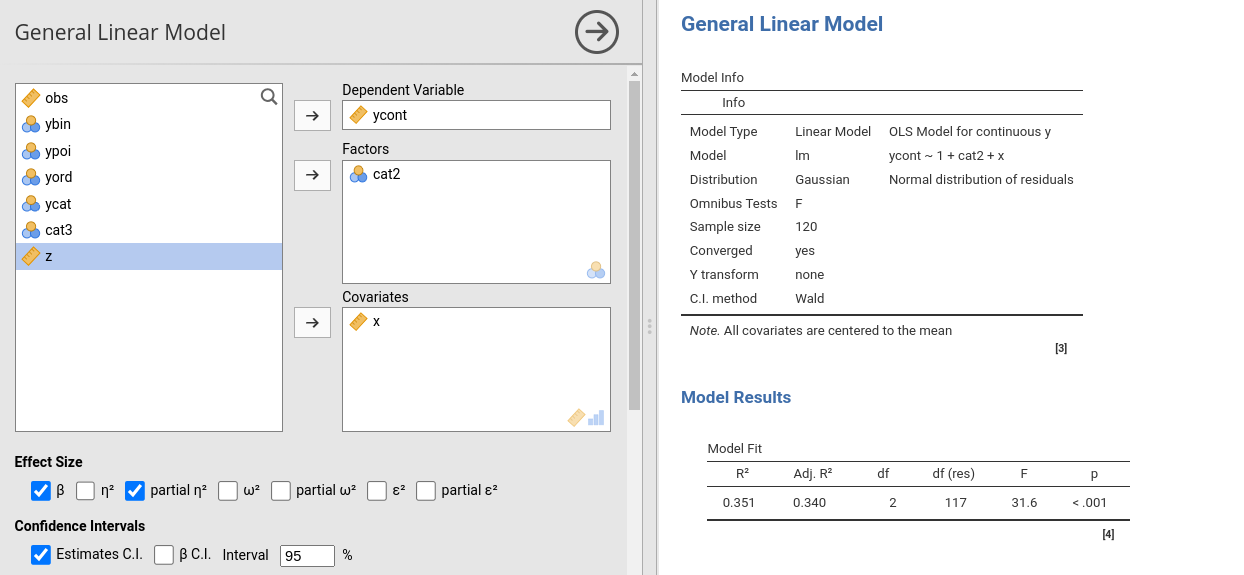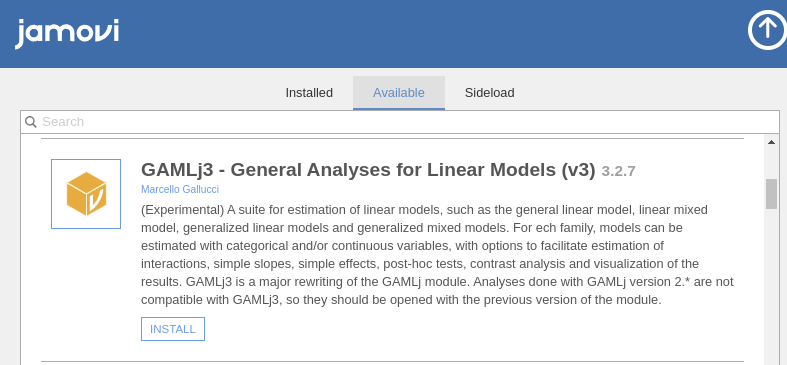General, Mixed and Generalized Models module for jamoviGAMLj offers tools to estimate, visualize, and interpret General Linear Models, Mixed Linear Models and Generalized Linear Models with categorial and/or continuous variables, with options to facilitate estimation of interactions, simple slopes, simple effects, post-hoc tests, etc.

• ANOVA and Regression approach
• Continuous and categorical independent variables
• F-test and parameter estimates
• Confidence intervals
• Simple slopes analysis
• Simple effects
• post-hocs analysis
• Plots for up to three-way interactions
• Automatic selection of best estimation methods and degrees of freedom selection
• Type III estimation

Available models are:

• OLS Regression (GLM)
• OLS ANOVA (GLM)
• OLS ANCOVA (GLM)
• Random coefficients regression (Mixed)
• Random coefficients ANOVA-ANCOVA (Mixed)
• Logistic regression (GZLM)
• Logistic ANOVA-like model (GZLM)
• Probit regression (GZLM)
• Probit ANOVA-like model (GZLM)
• Multinomial regression (GZLM)
• Multinomial ANOVA-like model (GZLM)
• Poisson regression (GZLM)
• Poisson ANOVA-like model (GZLM)
• Overdispersed Poisson regression (GZLM)
• Overdispersed Poisson ANOVA-like model (GZLM)
• Negative binomial regression (GZLM)
• Negative binomail ANOVA-like model (GZLM)
• Random coefficients logistic model (GlmMixed)
• Random coefficients poisson model (GlmMixed)

Please visit the sub-modules pages for details:

# Examples

Some worked out examples of the analyses carried out with jamovi GAMLj are posted here (more to come)

General Linear Model
Mixed models
Generalized Linear Models

# Installation

If you didn’t already, install Jamovi and run it. Select the jamovi modules library and install GAMLj from there## Others

You can clone this repository and compile the module within R with

library(jmvtools)

jmvtools::install()


# Install in R

To install it in R, simple use:


devtools::install_github("gamlj/gamlj")


# Troubleshooting

Please check out the troubleshooting page.

# Release notes

Please check out the Release notes.

# Quality control

Please check the rosetta store for alignment with other software results and possible discrepancies.